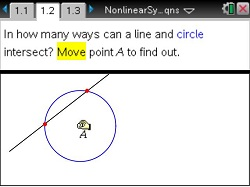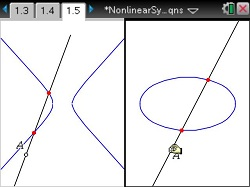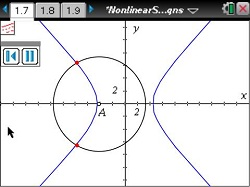# Activities

••• ##### Subject Area

• Math: Precalculus: Analytic Geometry (Conics)
• Math: College Algebra: Linear Systems and Matrices

• ##### Author9-12

45 Minutes

• ##### Device
•TI-Nspire™ CX/CX II
•TI-Nspire™ CX CAS/CX II CAS
• TI-Nspire™ Navigator™
• TI-Nspire™
• TI-Nspire™ CAS
• ##### Software

TI-Nspire™
TI-Nspire™ CAS

3.0

## Nonlinear Systems of Equations

#### Activity Overview

Students will be introduced to nonlinear systems of equations. It begins by allowing students to move figures around the screen to see ways certain types of graphs (linear/conic and conic/conic) can intersect each other and how many possible intersection points are possible. The activity concludes by having students look at the equations in a nonlinear system, stating how many solutions are possible, and then solving the system by graphing.

#### Key Steps

•In problem 1, students explore the number of possible intersection points when using nonlinear system of equations. They move a circle and observe how many ways a line intersects it.

•They move onto a hyperbola and an ellipse to find the number of possible intersection points.
Students may conjecture that for the graphs of a linear and quadratic function, there are either 0, 1, or 2 points of intersection.

•Students will look at intersections of a pair of graphs of quadratic functions. Students will see a circle and hyperbola with no intersection points. Here, they animate point A and watch the circle move across the screen. Students will see the circle intersect the hyperbola at 2, 3, and 4 places.